# Organic Chemistry Text Book (CHEM 3401 and 3402)

## 3.1 Bronsted Acids and Bases

### Acidity and Basicity

It is useful to begin a discussion of organic chemical reactions with a review of acid-base chemistry and terminology for several reasons. First, acid-base reactions are among the simplest to recognize and understand. Second, some classes of organic compounds have distinctly acidic properties, and some other classes behave as bases, so we need to identify these aspects of their chemistry. Finally, many organic reactions are catalyzed by acids and/or bases, and although such transformations may seem complex, our understanding of how they occur often begins with the functioning of the catalyst.
Organic chemists use two acid-base theories for interpreting and planning their work: the Brønsted theory and the Lewis theory

#### Brønsted Theory

According to the Brønsted theory, an acid is a proton donor, and a base is a proton acceptor. In an acid-base reaction, each side of the equilibrium has an acid and a base reactant or product, and these may be neutral species or ions.

 H-A   +   B:(–)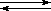A:(–)   +   B-H (acid1)   (base1) (base2)   (acid2)

Structurally related acid-base pairs, such as {H-A and A:(–)} or {B:(–) and B-H} are called conjugate pairs. Substances that can serve as both acids and bases, such as water, are termed amphoteric.

 H-Cl   +   H2OCl:(–)   +   H3O(+) (acid)     (base) (base)     (acid)

 H3N:   +   H2ONH4(+)   +   HO(–) (base)     (acid) (acid)       (base)

The relative strength of a group of acids (or bases) may be evaluated by measuring the extent of reaction that each group member undergoes with a common base (or acid). Water serves nicely as the common base or acid for such determinations. Thus, for an acid H-A, its strength is proportional to the extent of its reaction with the base water, which is given by the equilibrium constant Keq.

 H-A   +   H2OH3O(+)   +  A:(–)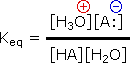Since these studies are generally extrapolated to high dilution, the molar concentration of water (55.5) is constant and may be eliminated from the denominator. The resulting K value is called the acidity constant, Ka. Clearly, strong acids have larger Ka's than do weaker acids. Because of the very large range of acid strengths (greater than 1040), a logarithmic scale of acidity (pKa) is normally employed. Stronger acids have smaller or more negative pKa values than do weaker acids.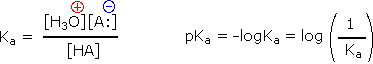Some useful principles of acid-base reactions are:
• The stronger the acid the weaker its conjugate base; the stronger the base the weaker its conjugate acid.
• Acid-base equilibria always favor the weakest acid and the weakest base.

#### Examples of Brønsted Acid-Base Equilibria

Acid-Base Reaction   Conjugate
Acids
Conjugate
Bases
Ka pKa
HBr   +   H2O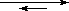H3O(+)   +   Br(–) HBr
H3O(+)
Br(–)
H2O
105 -5
CH3CO2H   +   H2O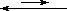H3O(+)   +   CH3CO2(–) CH3CO2H
H3O(+)
CH3CO2(–)
H2O
1.77*10-5 4.75
C2H5OH   +   H2OH3O(+)   +   C2H5O(–) C2H5OH
H3O(+)
C2H5O(–)
H2O
10-16 16
NH3   +   H2OH3O(+)   +   NH2(–) NH3
H3O(+)
NH2(–)
H2O
10-34 34

In all the above examples water acts as a common base. The last example ( NH3 ) cannot be measured directly in water, since the strongest base that can exist in this solvent is hydroxide ion. Consequently, the value reported here is extrapolated from measurements in much less acidic solvents, such as acetonitrile.

Since many organic reactions either take place in aqueous environments ( living cells ), or are quenched or worked-up in water, it is important to consider how a conjugate acid-base equilibrium mixture changes with pH. A simple relationship known as the Henderson-Hasselbalch equation provides this information.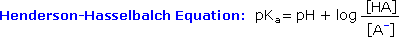When the pH of an aqueous solution or mixture is equal to the pKa of an acidic component, the concentrations of the acid and base conjugate forms must be equal ( the log of 1 is 0 ). If the pH is lowered by two or more units relative to the pKa, the acid concentration will be greater than 99%. On the other hand, if the pH ( relative to pKa ) is raised by two or more units the conjugate base concentration will be over 99%. Consequently, mixtures of acidic and non-acidic compounds are easily separated by adjusting the pH of the water component in a two phase solvent extraction.
For example, if a solution of benzoic acid ( pKa = 4.2 ) in benzyl alcohol ( pKa = 15 ) is dissolved in ether and shaken with an excess of 0.1 N sodium hydroxide ( pH = 13 ), the acid is completely converted to its water soluble ( ether insoluble ) sodium salt, while the alcohol is unaffected. The ether solution of the alcohol may then be separated from the water layer, and pure alcohol recovered by distillation of the volatile ether solvent. The pH of the water solution of sodium benzoate may then be lowered to 1.0 by addition of hydrochloric acid, at which point pure benzoic acid crystallizes, and may be isolated by filtration.

For a discussion of how acidity is influenced by molecular structure Click Here.

Basicity

The basicity of oxygen, nitrogen, sulfur and phosphorus compounds or ions may be treated in an analogous fashion. Thus, we may write base-acid equilibria, which define a Kb and a corresponding pKb. However, a more common procedure is to report the acidities of the conjugate acids of the bases ( these conjugate acids are often "onium" cations ). The pKa's reported for bases in this system are proportional to the base strength of the base. A useful rule here is:  pKa + pKb = 14.
We see this relationship in the following two equilibria:

Acid-Base Reaction   Conjugate
Acids
Conjugate
Bases
K pK
NH3   +   H2ONH4(+)   +   OH(–) NH4(+)
H2O
NH3
OH(–)
Kb = 1.8*10-5 pKb = 4.74
NH4(+)   +   H2OH3O(+)   +   NH3 NH4(+)
H3O(+)
NH3
H2O
Ka = 5.5*10-10 pKa = 9.25

Tables of pKa values for inorganic and organic acids ( and bases) are available in many reference books, and may be examined here by clicking on the appropriate link:

Although it is convenient and informative to express pKa values for a common solvent system (usually water), there are serious limitations for very strong and very weak acids. Thus acids that are stronger than the hydronium cation, H3O(+), and weak acids having conjugate bases stronger than hydroxide anion, OH(–), cannot be measured directly in water solution. Solvents such as acetic acid, acetonitrile and nitromethane are often used for studying very strong acids. Relative acidity measurements in these solvents may be extrapolated to water. Likewise, very weakly acidic solvents such as DMSO, acetonitrile, toluene, amines and ammonia may be used to study the acidities of very weak acids. For both these groups, the reported pKa values extrapolated to water are approximate, and many have large uncertainties.  A useful table of pKa values in DMSO solution has been compiled from the work of F.G. Bordwell, and may be reached by Clicking Here.